# Python同步遍历多个列表

Python的for循环十分灵活，使用for循环我们可以很轻松地遍历一个列表，例如：

a_list = ['z', 'c', 1, 5, 'm']
for each in a_list:
print(each)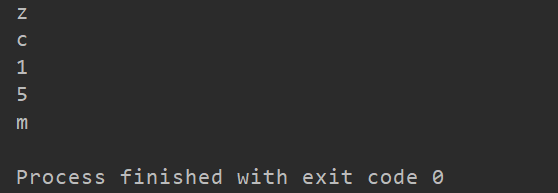## ①使用zip()函数 （推荐）

name_list = ['张三', '李四', '王五']
age_list = [54, 18, 34]
for name, age in zip(name_list, age_list):
print(name, ':', age)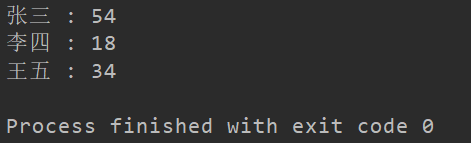name_list = ['张三', '李四', '王五']
age_list = [54, 18, 34]
print(zip(name_list, age_list))
print(type(zip(name_list, age_list)))
print(*zip(name_list, age_list))
print(list(zip(name_list, age_list)))
print(dict(zip(name_list, age_list)))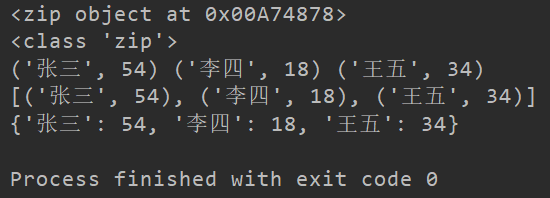list1 = [1, 2, 3, 4, 5]
list2 = ['a', 'b', 'c', 'd', 'f']
list3 = ['A', 'B', 'C', 'D', 'F']

for number, lowercase, capital in zip(list1, list2, list3):
print(number, lowercase, capital)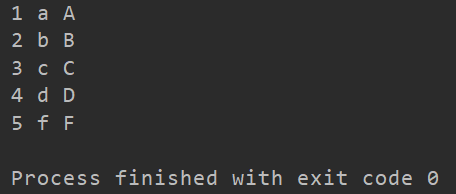## ②利用下标

list1 = [1, 2, 3, 4, 5]
list2 = ['a', 'b', 'c', 'd', 'f']

n = 0
for each in list1:
print(each, list2[n])
n += 1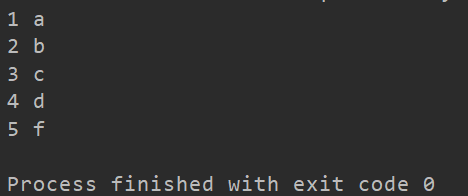10-061万+
11-122万+
10-214673
09-241万+
11-141万+
03-10254
09-062947
06-205万+
05-054575